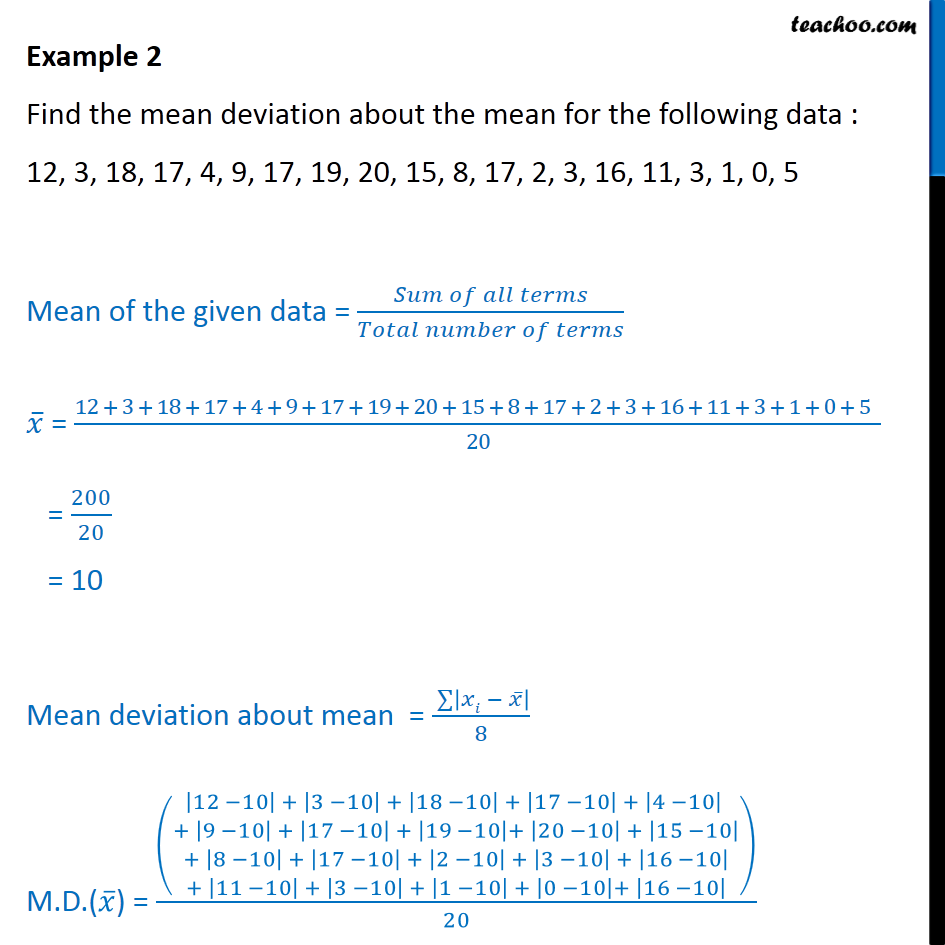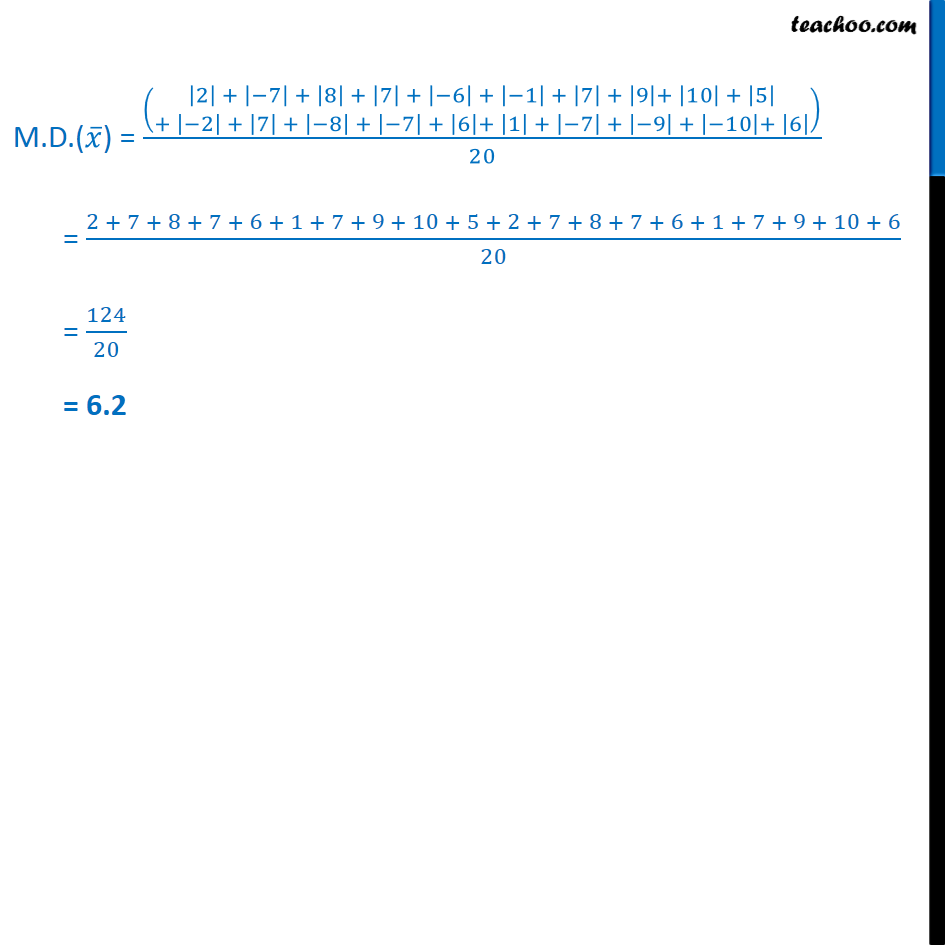Mean deviation about mean - Ungrouped

Chapter 13 Class 11 Statistics
Concept wiseLearn in your speed, with individual attention - Teachoo Maths 1-on-1 Class

### Transcript

Example 2 Find the mean deviation about the mean for the following data : 12, 3, 18, 17, 4, 9, 17, 19, 20, 15, 8, 17, 2, 3, 16, 11, 3, 1, 0, 5 Mean of the given data = = 12 + 3 + 18 + 17 + 4 + 9 + 17 + 19 + 20 + 15 + 8 + 17 + 2 + 3 + 16 + 11 + 3 + 1 + 0 + 5 20 = 200 20 = 10 Mean deviation about mean = 8 M.D.( ) = 12 10 + 3 10 + 18 10 + 17 10 + 4 10 + 9 10 + 17 10 + 19 10 + 20 10 + 15 10 + 8 10 + 17 10 + 2 10 + 3 10 + 16 10 + 11 10 + 3 10 + 1 10 + 0 10 + 16 10 20 M.D.( ) = 2 + 7 + 8 + 7 + 6 + 1 + 7 + 9 + 10 + 5 + 2 + 7 + 8 + 7 + 6 + 1 + 7 + 9 + 10 + 6 20 = 2 + 7 + 8 + 7 + 6 + 1 + 7 + 9 + 10 + 5 + 2 + 7 + 8 + 7 + 6 + 1 + 7 + 9 + 10 + 6 20 = 124 20 = 6.2